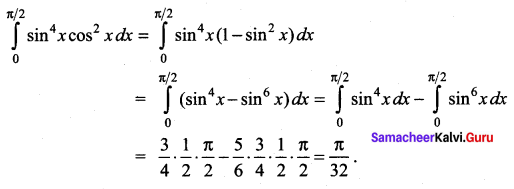# Samacheer Kalvi 12th Maths Solutions Chapter 9 Applications of Integration Ex 9.6

## Tamilnadu Samacheer Kalvi 12th Maths Solutions Chapter 9 Applications of Integration Ex 9.6

Question 1.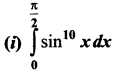Solution: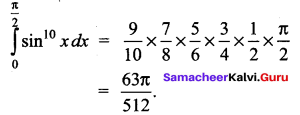(ii)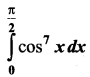Solution: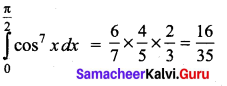(iii)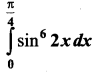Solution: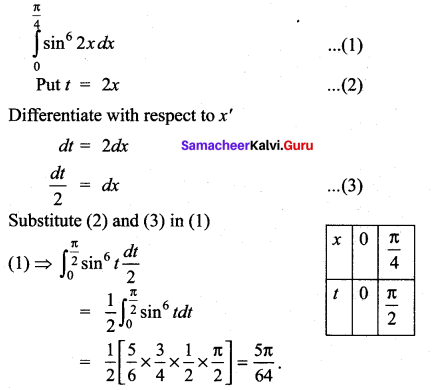(iv)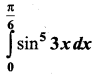Solution: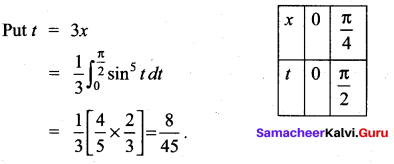(v)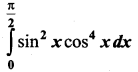Solution: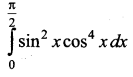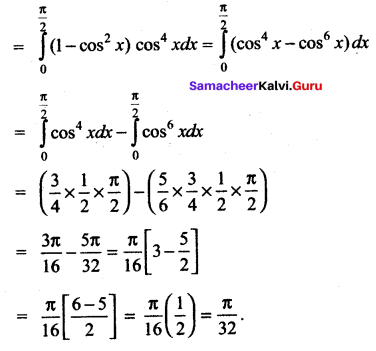(vi)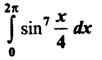Solution: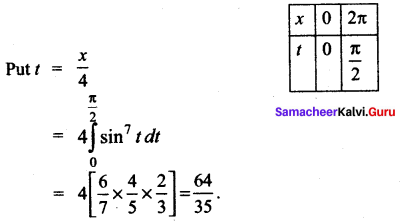(vii)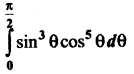Solution:
We know that,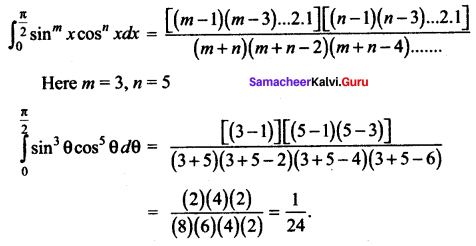(viii)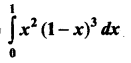Solution: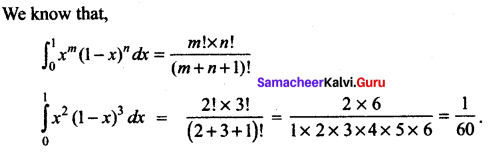### Samacheer Kalvi 12th Maths Solutions Chapter 9 Applications of Integration Ex 9.6 Additional Problems

Question 1.
Evaluate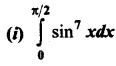Solution: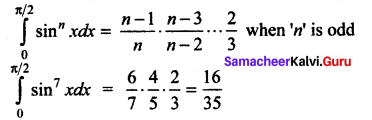(ii)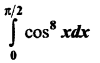Solution: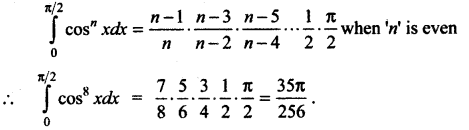(iii)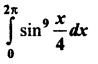Solution: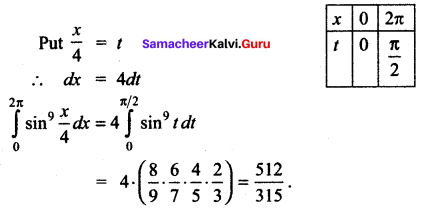(iv)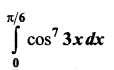Solution: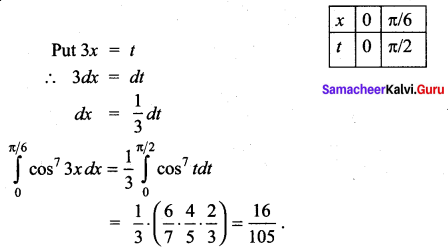Question 2.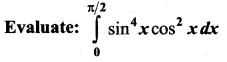Solution: